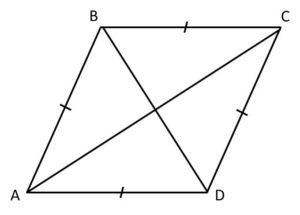# Java Program To Calculate Area Of Rhombus | 4 Ways

Java program to find the area of a rhombus or calculate the area of a rhombus. We also added the online compiler and execution tool in order to check the program for your values. There were so many methods to find/calculate.

What is a rhombus?

A rhombus is a flat shaped, which have equal in length of all sides and opposite sides are equal and the angles too.What is the formula?

There may be so many methods to find the area of a rhombus. Here we share the simple formula. Check it out.

( Diagonal(D1)*Diagonal(D2)/2 )

Here is the sample program method -1 # Online execution tool # java program to find the area of rhombus #

Output :

Online Execution Tool for the above rhombus code :

How simple it was right? If you were new to java programming and have no idea how the above program works, then check out the below guide about java program.

Code explanation :

– Which reads the input values from the main system and submit them to the main block code or consider it has a pillar for the program. The main block scanner function will use the main Methods from JDK package.

– It’s a blueprint, where objects are created within a class.

– The scanner is a method, which reads the input values from the system. And, the system.in is a function which can read the values from your system( import.java.util.Scanner).

– Syntax to represent the output values.

– It is a method, which takes a string as an argument and converts into the double format. Where D1 is named for memory space name, where you store the values. Nextdouble(); the values store and return to next function.same as follows for the rest of the code too.

– The java formula.

– Output displayed here.

That’s the complete explanation of the above code. For a subject purpose, we have shared the two more examples here check it out.

Sample Method – 2 #

Here is another simple method to calculate # Sample Method – 2#

Note: The following program is just for a subject purpose. Because we are directly dumping the variables into the program, such that there is no need to give the input variables on the cmd screen #Justforsubjectpurpose#

Output :

What is the difference between ” Int ” and ” Double”?

If you check out compare the sample code #1 and sample code #2. We have changed the data variables. The only difference is where the double can use to represent the values in decimals points to (135.0), whereas in Int, only the integer values will be displayed on the screen too( 135).

Sample method # 3 :

What if you don’t know the value of diagonal, then how can you calculate the area. Well, here is the method to find out the diagonal.

A rhombus is nothing but the combination of two triangles. If you know the value of one length, then you can know the value of another length since the opposite sides are parallel.

Once, you know the value of a side. Then you can find out the diagonal with the help of hypotenuse. And, now find out the area of a triangle of two triangles, and then sum-it-up to get the area.

x

## X Star Pattern Java Program – Patterns

Java program to print X star pattern program – We have written the below print/draw ...